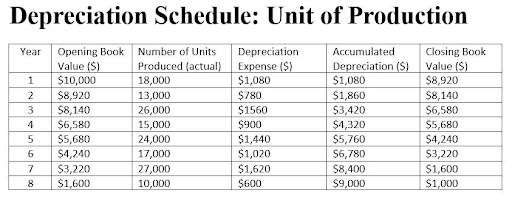## Instead of calculating each ratio individually

Let our ratio tool instantly calculate your ratios. Input your financial data once and get multiple results.

## Depreciation Schedule (Unit of Production Method)

Depreciation (unit of production method) =

(Cost of asset - Residual value)

Estimated total units expected

x Actual units produced

### INTERPRETATION

The unit of production method is used when the asset’s value is related to the number of units it produced instead of the years it was useful.

Business owners, managers, and other interested parties prefer this method for businesses that use machinery or equipment to make a product.

Note: expanded calculation
Divide the asset’s cost―less its residual, aka salvage value―by the total units you expect the asset to produce over its useful life. Then, multiply this rate by the actual units produced during the year.

### EXAMPLE

M&M purchased a machine for \$10,000, the residual value is \$1000, and the machine is expected to be useful for 150,000 units. In year 1, M&M’s machine produced 18000 units, and 13000 units in year 2 (see chart for consecutive years).

Description of asset: Labeling Machine
Year (start): 20XX
Residual value (salvage value): \$1,000
Useful Units (estimated total units expected): 150,000
Depreciation per unit: \$0.06
Depreciable Cost: \$9,000

### SEE: Depreciation Schedule - Unit of Production Method

Depreciable Cost = cost of asset – residual value
Depreciable Cost = \$10,000 – \$1,000 = \$9,000

Depreciation per Unit = Depreciable cost / Useful units
Depreciation per Unit = \$9,000 / 150,000 = \$0.06

Depreciation Expense = Depreciation per Unit x Number of Units Produced
Depreciation Expense = \$0.06 x 18,000 units = \$1,080

Accumulated Depreciation = Depreciation Expense + Accumulated Depreciation
Accumulated Depreciation (year 2) = \$1,080 + \$780 = \$1,860

Closing Book Value = Opening Book Value – Depreciation Expense
Closing Book Value = \$10,000 – \$1,080 = \$8,920

### BENCHMARK: ROT

Companies that use machinery or equipment to make a product apply this method because it provides a more accurate picture of profits and losses by spreading the cost of such assets over the years based on usage.Depreciation (unit of production method):

### ABBREVIATION KEY:

ROT: Rule of thumb
HA: Historical Average (organization’s historical average)
PG: Peer Group average
EB: Economic Benchmark

DISCLAIMER: The interactive calculators on this site are self-help tools intended to help you visualize and explore your financial information. They are not intended to replace the advice of a qualified professional. Because each business is different, we can not guarantee accuracy.